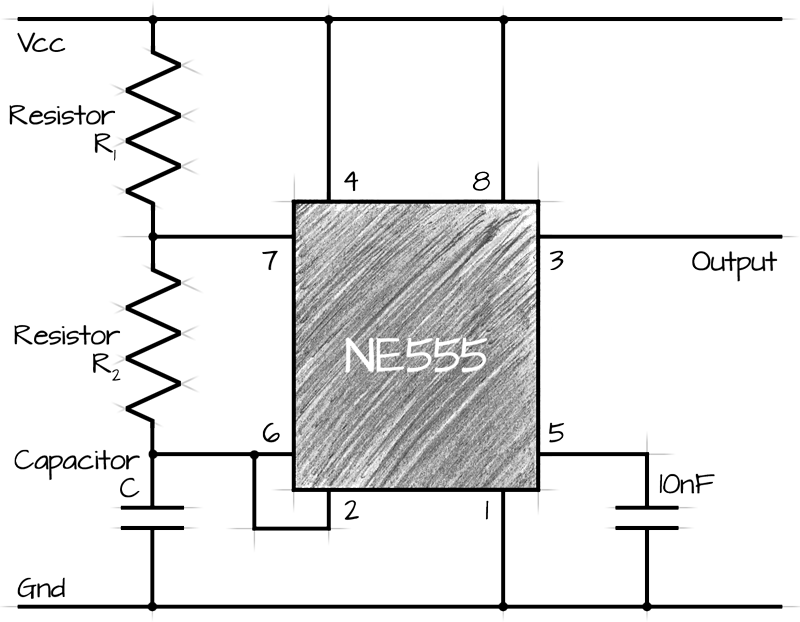# 555 Astable Circuit Calculator

The 555 timer is capable of being used in astable and monostable circuits. In an astable circuit, the output voltage alternates between VCC and 0 volts on a continual basis.By selecting values for R1, R2 and C we can determine the period/frequency and the duty cycle.

The period is the length of time it takes for the on/off cyle to repeat itself, whilst the duty cycle is the percentage of time the output is on. ie. T1/T.

In this type of circuit, the duty cycle can never be 50% or lower.Notes:
• Increasing C will increase the cycle time (and hence, reduce the frequency).
• Increasing R1 will increase Time High (T1), but will leave Time Low (T0) unaffected.
• Increasing R2 will increase Time High (T1), increase Time Low (T0) and decrease the duty cycle (down to a minimum of 50%)